## Tuesday, January 01, 20192015
(May)
MATHEMATICS
(Major)
Course: 401
[A: Computer Programming (C Programming)]
Full Marks: 50
Pass Marks: 20
Time: 2 ½ hours
The figures in the margin indicate full marks for the questions

1. Choose the correct answer: 1x5=5
1. Which of the following is not a character constant in C?
1. ‘Thank you’
2. ‘Enter values of P, N, R’
3. ’23.5 E-03’
4. All of the above
1. Which of the following statements is wrong in C?
1. Mes = 123.56;
2. Con = T *A;
3. This = T * 20;
4. 3 + a = b
1. If an integer is to be entered through the keyboard, which function would you use?
1. Scanf ()
2. Gets ()
3. Getche ()
4. Getchar ()
1. Which of the following statements is used to take the control in the beginning of a loop?
1. Exit.
2. Break.
3. Continue.
4. None of the above.
1. The array int num has
1. Twenty-six elements.
2. Twenty-five elements.
3. Twenty-seven elements.
4. Twenty-four elements.
2. Answer any eight of the following questions: 2x8=16
1. Draw the flowchart of finding the largest number among three numbers.
2. The radius of a circle is input through the keyboard. Write a C program to calculate the area of the circle.
3. Write a short note on algorithm.
4. Mention the bitwise operators in C.
5. Evaluate the following expression assuming that k is a float variable:
K = 3/2 * 4 + 3/ 8 + 3
1. State the errors, if any, in the following input statements:
1. Scanf (“%c%f%d”, city, &price, &year);
2. Scanf (“%s%d”, city, amount);
1. Write a C program to print the odd integers between 1 and 49.
2. State the errors, if any, if the following function declarations:
1. Int sum (int a, b);
2. Int (fun) void;
1. Write the syntax of the ‘simple if statement’ and draw flowchart for it.
2. Write a C program to determine whether an integer is divisible by 3 or not.
3. Convert the equation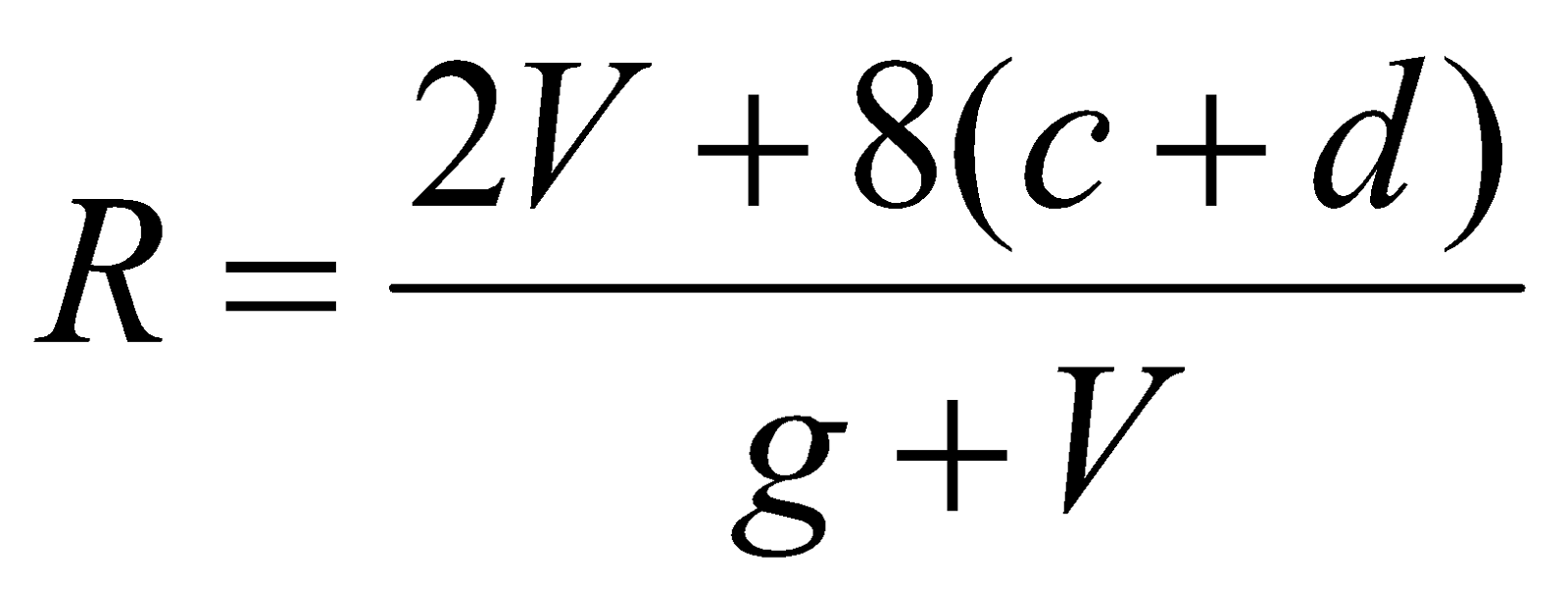into corresponding C statement.
3. Write a C program to determine the area of a triangle using the formula, area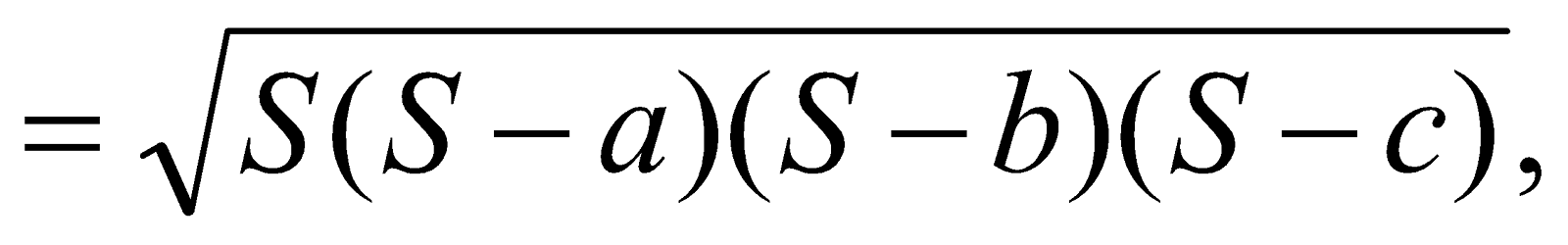where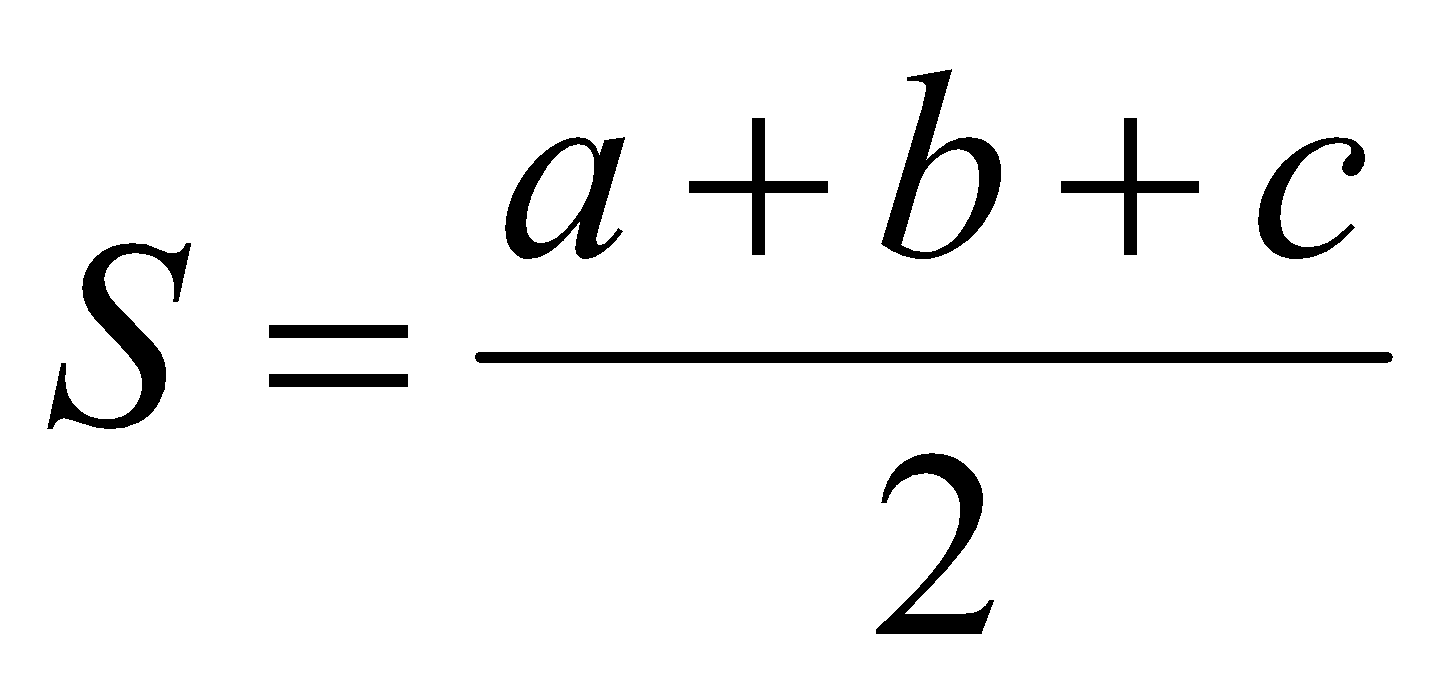Or
Write a C program to interchange the contents of two variables.
4. Write a C program to read the values of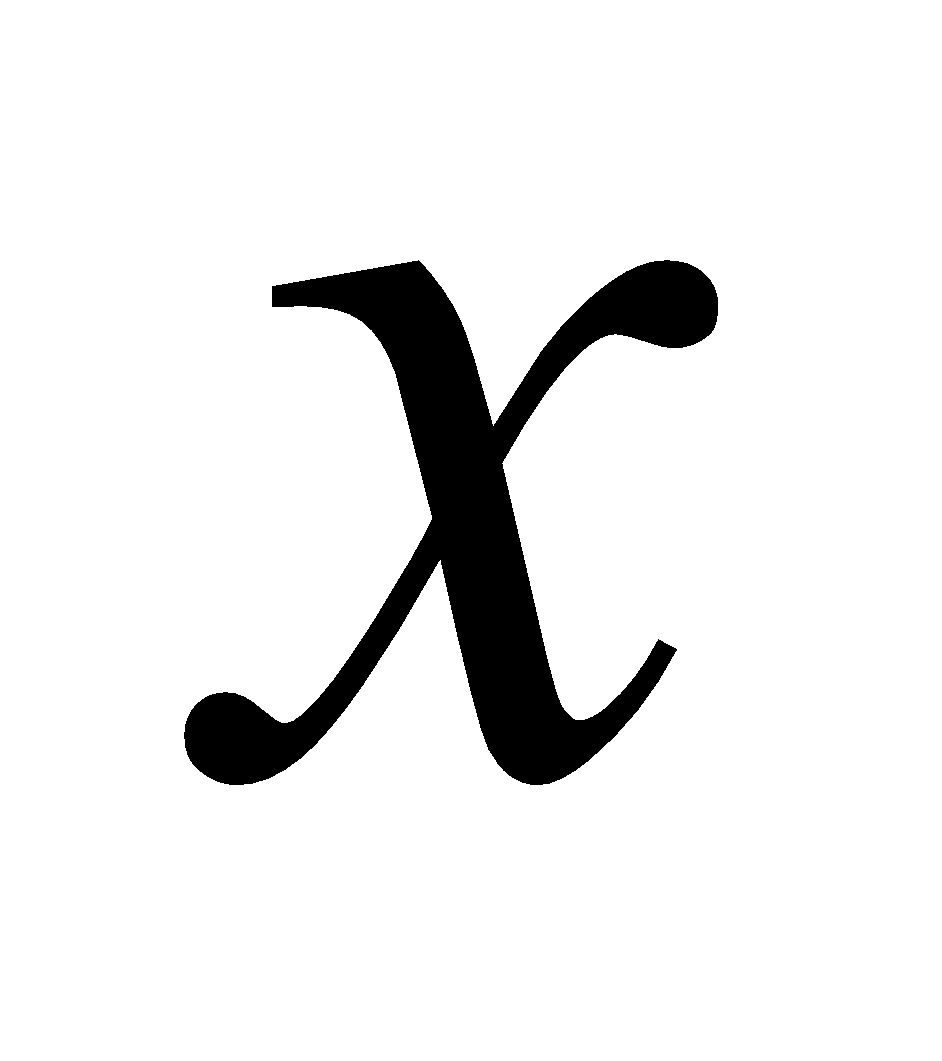and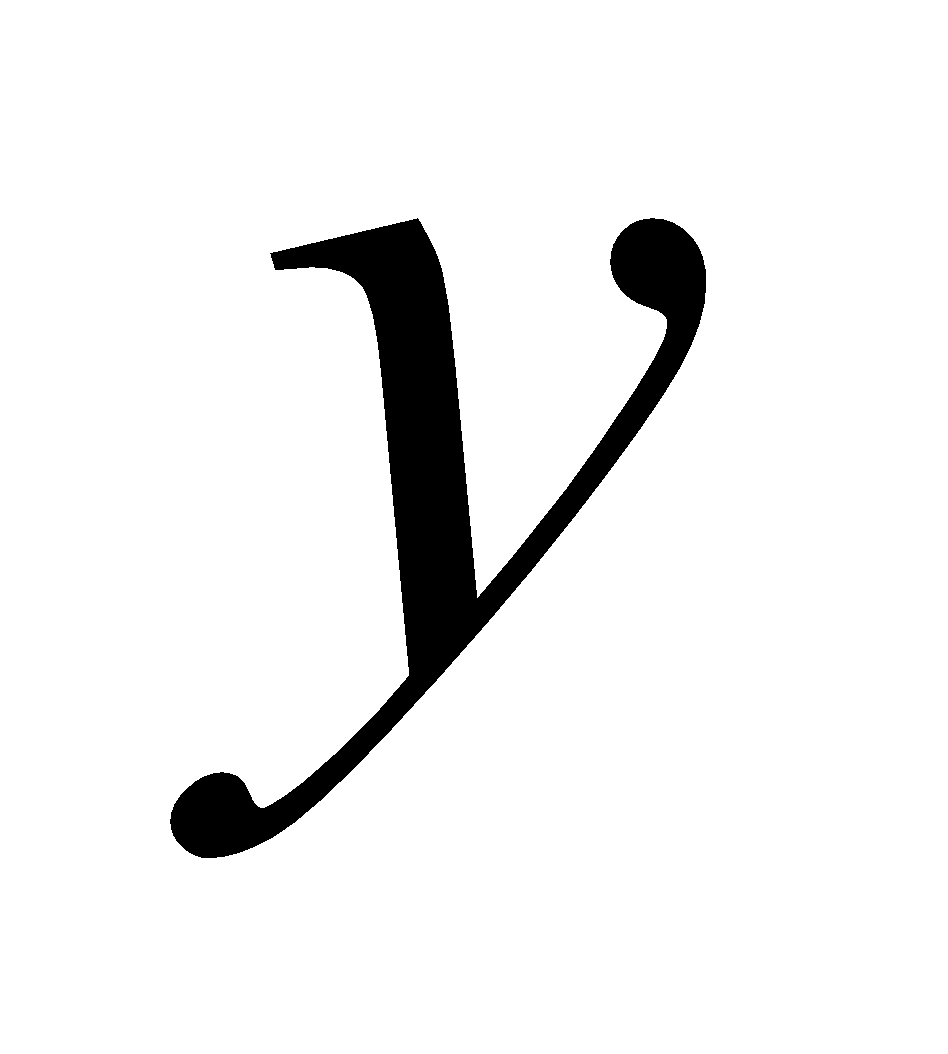, and print the result of the expression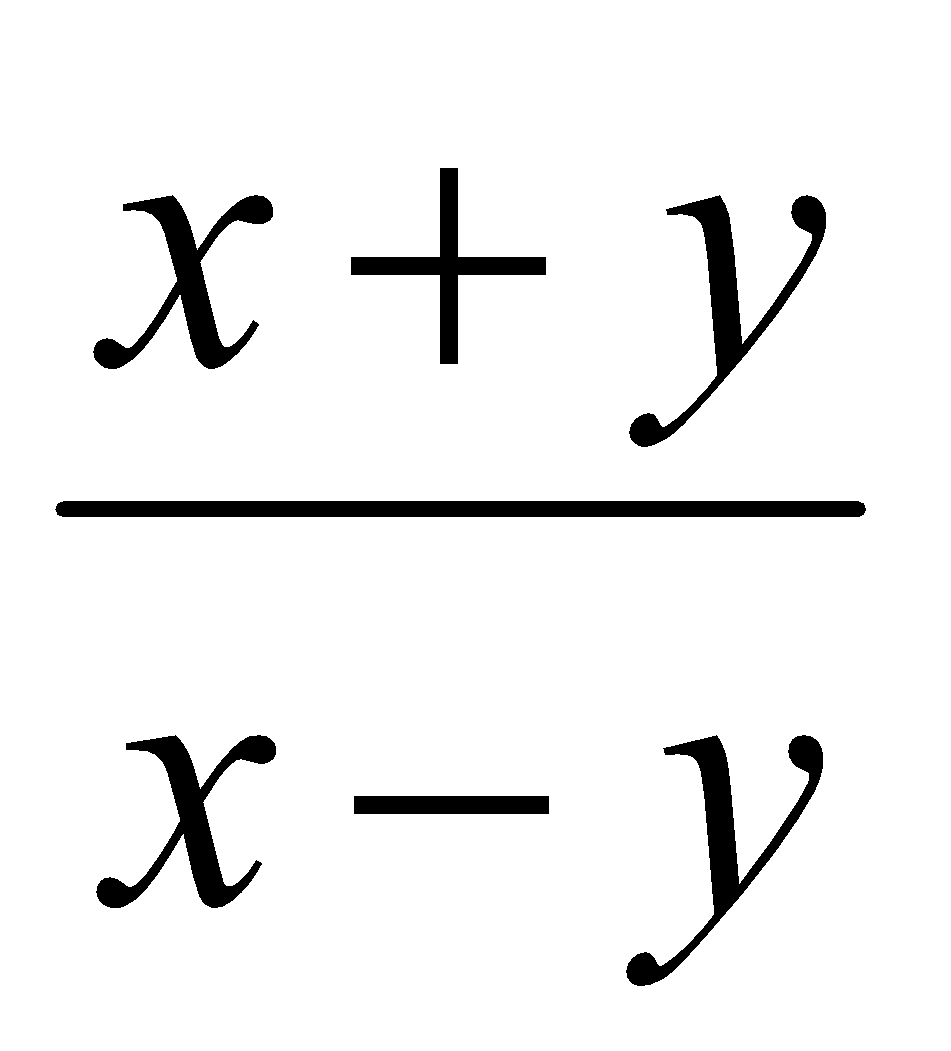3
Or
Write a C program to evaluate the sum of squares of all integers between 1 and 10.
5. If the marks obtained by a student in 5 different subjects are input through the keyboard, write a C program to find out the aggregate marks and percentage marks obtained by the student. Assume that the maximum marks that can be obtained by a student in each subject is 100.
Or
Write a C program to compute compound interest.
6. In a company, an employee is paid as under: If his basic salary is less than Rs. 1,500, then house rent allowance (HRA) is 10% of basic salary and dearness allowance (DA) is 90% of basic salary. If his basic salary is either equal to or above Rs. 1,500, then HRA = Rs. 500 and DA = 98% of the basic salary.
If the employee’s salary is input through the keyboard, write a C program to find his gross salary. 4
Or
A year is input through the keyboard. Write a C program to determine whether the year is a leap year or not.
7. What is an array? Write a C program to choose the maximum of a one-dimensional array of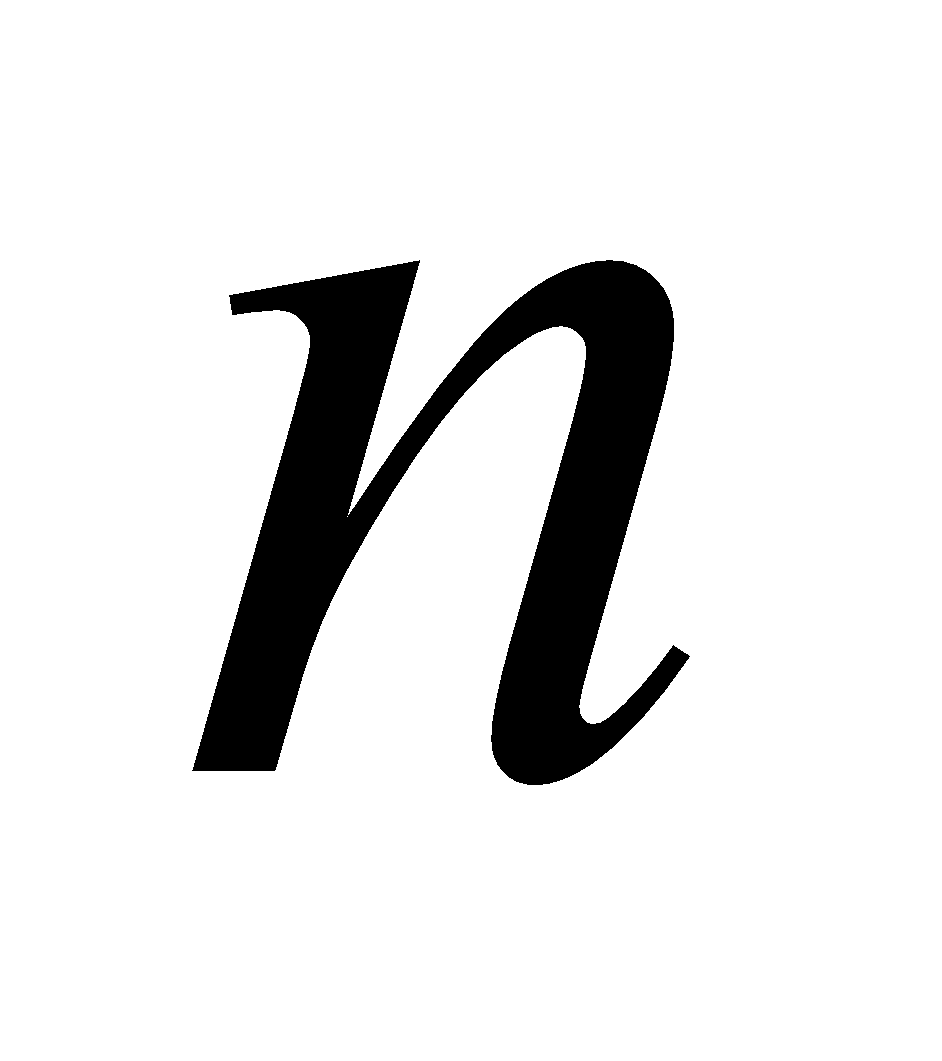numbers.    1+3=4
Or
Write a C program to determine whether a number is prime or not. 4
8. Write a C program to find the transpose of a matrix of order    3 x 3. 3
Or
Write a C program to evaluate the determinant of a matrix of order    2 x 2.
9. What is user defined function? Write a C program to evaluate the cube of an integer using function prototype. 1+3=4
Or
Write a C program to print all Fibonacci numbers less than 100.
10. Answer any one of the following: 4
1. Write a C program to find the sum of cosine series up to 10 terms.
2. Write a C program to add two matrices or order 3 x 3.
3. Write a C program to compute the roots of the quadratic equation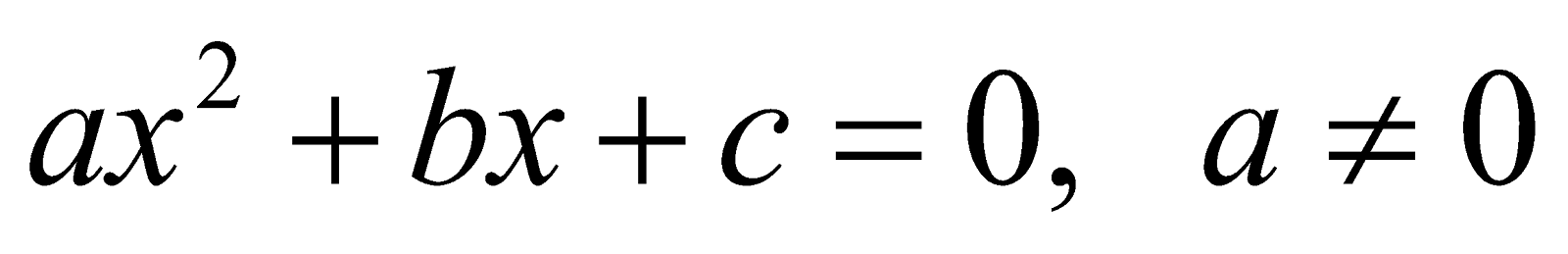***